Updated: 11/28/2020

# Evaluating Diagnostic Tests

0%
Topic
Review Topic
0
0
N/A
N/A
Questions
11
0
0
Topic
 Sensitivity, Specificity, PPV, NPV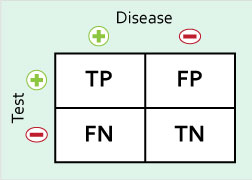These 4 measures describe how well diagnostic tests capture the true presence or absence of disease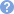Sensitivity (SN) % with disease who test positive = a/(a+c) = TP/(TP+FN) Specificity (SP) % without disease who test negative = d/(b+d) = TN/(FP+TN) Positive predictive value (PPV)% positive test results that are true positives = a/(a+b) = TP/(TP+FP)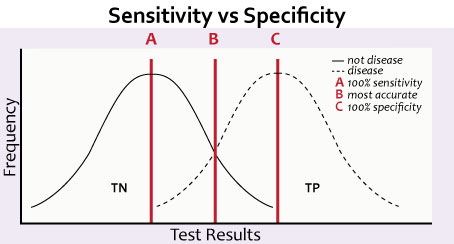Negative predictive value (NPV)% negative test results that are true negatives = d/(c+d) = TN/(FN+TN) Cut-off point may be adjusted to optimize sensitivity and specificity, which are inversely related (cut-off point with decreased sensitivity is associated with increased specificity and vice-versa)will also affect NPV and PPVi.e., decrease in sensitivity associated with decrease in NPV in the same population (due to higher false negative rates) Sensitivity and specificity are intrinsic to the diagnostic testdo not change with prevalence PPV and NPV do change with prevalenceReceiver operating characteristic (ROC) curves are a graphical depiction of a test's performance Y axis: sensitivity X axis: 1-specificity The higher the curve, the better the test This is quantified by the AUC (area under the curve); an AUC of 0.5 states that the test performs no better than chance (bad test!), whereas an AUC of 0.9 suggests a better-performing test
 Odds Ratio, Relative Risk, Attributable Risk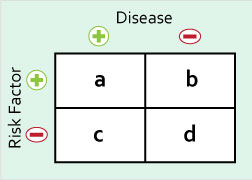These measures describe the relationship between a risk factor and a disease Odds Ratio (OR) odds of having disease in expose group / odds of having disease in unexposed group = ad/bc Relative Risk (RR) probability of getting disease in exposed group / probability of getting disease in unexposed group = [a/(a+b)] / [c/(c+d)] If RR = 1, there is no association between exposure and outcomeDose-reponse increased level of exposure shows an increased relative risk of developing/odds ratio of having a disease can be used in OR or RR to support causalityAttributable Risk (AR) risk in exposed group - risk in unexposed group = a/(a+b) - c/(c+d)

Average 5.0 of 1 Ratings

Questions (11)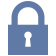Sorry, this question is forSorry, this question is for

(M2.OMB.18.1) Health officials are considering a change be made to the interpretation of the tuberculin skin test that will change the cut-off for a positive purified protein derivative (PPD) from 10 mm to 15 mm for healthcare workers. Which of the following can be expected as a result of this change?

QID: 210446
1

Decrease the sensitivity

30%

(7/23)

2

Decrease the specificity

17%

(4/23)

3

Increase the precision

0%

(0/23)

4

Increase the sensitivity

35%

(8/23)

5

No change to the sensitivity or specificity

9%

(2/23)

M 5 C

Select Answer to see Preferred ResponseSorry, this question is forSorry, this question is for

(M2.OMB.18.1) A home drug screening test kit is currently being developed. The cut-off level is initially set at 4 mg/uL, which is associated with a sensitivity of 92% and a specificity of 97%. How might the sensitivity and specificity of the test change if the cut-off level is changed to 2 mg/uL?

QID: 210448
1

Sensitivity = 90%, specificity = 99%

12%

(1/8)

2

Sensitivity = 92%, specificity = 97%

25%

(2/8)

3

Sensitivity = 95%, specificity = 98%

12%

(1/8)

4

Sensitivity = 97%, specificity = 96%

50%

(4/8)

5

Sensitivity = 100%, specificity = 97%

0%

(0/8)

M 5 C

Select Answer to see Preferred ResponseSorry, this question is forSorry, this question is for